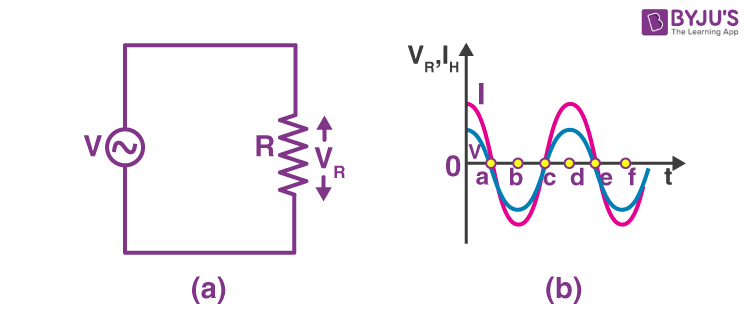Checkout JEE MAINS 2022 Question Paper Analysis : Checkout JEE MAINS 2022 Question Paper Analysis :

# AC Voltage Applied to a Resistor

In this section, we will deal with an electric circuit, where an AC voltage is applied across a resistor. Before applying AC voltage across the resistor, let us know what an alternating current (AC) is. Electric current, which reverses its direction periodically and changes its magnitude continuously with time, is known as an alternating current.

## AC Voltage Applied to a Resistor Derivation

Let us consider the circuit as shown below. We have a resistor and an AC voltage V, represented by the symbol ~, that produces a potential difference across its terminals that varies sinusoidally. Here, the potential difference or the AC voltage can be given as

$$\begin{array}{l}v=v_{m}\; sin\; \omega t\end{array}$$

Here, vm is the amplitude of the oscillating potential difference and the angular frequency is given by ω.The current through the resistor due to the present voltage source can be calculated using Kirchhoff’s loop rule, as under,

$$\begin{array}{l}\sum V(t)=0\end{array}$$

Here, using this equation, we can write,

$$\begin{array}{l}v_{m}\; sin\; \omega t=iR\end{array}$$

Or,

$$\begin{array}{l}i=\frac{v_{m}}{R}\; sin\; \omega t\end{array}$$

Here, R is the resistance of the given resistor, and hence we can write,

$$\begin{array}{l}i=i_{m}\; sin\; \omega t\end{array}$$

From the Ohm’s law, we can write,

$$\begin{array}{l}i_{m}=\frac{v_{m}}{R}\end{array}$$

As it works equally well for AC voltages and DC voltages. We saw that the voltage across the resistor and the current passing through it are both sinusoidal quantities and are represented by the graph shown in the figure above. Both the quantities are in phase with each other.

The instantaneous power in the circuit is given by,

$$\begin{array}{l}P=i^{2}R=i_{m}^{2}R\; sin^{2}\; \omega t\end{array}$$

The average value of power over the complete cycle is given as,

$$\begin{array}{l}\bar{P}= i^{2}R =i_{m}^{2}R\; sin^{2}\; \omega t\end{array}$$

Here the quantities im and R are constants, and hence, the above equation is evaluated as,

$$\begin{array}{l}\bar{P}= i_{m}^{2}R sin^{2}\; \omega t\end{array}$$

Using trigonometry, we have

$$\begin{array}{l}sin^2 \omega t = \frac{1}{2}\;\;(1\;- \;cos \;2\omega t)\end{array}$$
, and we can write
$$\begin{array}{l}sin^2 \omega t = \frac{1}{2}\;\;(1\;- \;cos \;2\omega t)\end{array}$$
And since the integration of cos 2ωt over the complete cycle is zero. So we can write
$$\begin{array}{l}cos\;2ωt\; =\; 0\end{array}$$
, we can write,

$$\begin{array}{l} sin^{2}\; \omega t =\frac{1}{2}\end{array}$$

Thus we can also write,

$$\begin{array}{l}\bar{P}=\frac{1}{2}i_{m}^{2}R\end{array}$$

It is important to note that the AC power can also be expressed as DC power if we denote the current in terms of the effective current or the root-mean-square current, which is given as,

$$\begin{array}{l}I_{rms}=\sqrt{\bar{i^{2}}}=\sqrt{\frac{1}{2}i_{m}^{2}}=\frac{i_{m}}{\sqrt{2}}=0.707i_{m}\end{array}$$

• AC Voltage Applied to an Inductor
• AC Voltage Capacitor
• AC and DC Difference
• Click the below video to understand the difference between AC and DC:## Frequently Asked Questions – FAQs

### What is direct current?

The unidirectional flow of electric charges is known as direct current.

### Does AC change its magnitude continuously with time?

Yes, alternating current changes its magnitude continuously with time.

### Define frequency of the electrical signal.

The number of cycles per second is known as frequency. Frequency is measured in hertz.

Stay tuned with BYJU’S to learn more about the AC voltages, the behaviour of the circuit when AC voltage is applied across a resistor and much more.

Test your knowledge on AC voltage inductor